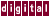## 7.3.2 Global Forward Substitution

A global forward substitution pass finds relations between variables that do not necessarily depend on the loop index variables, such as `n` and `np1` , as shown in the following example:

```np1 = n + 1;
for ( i=0; i<m; i++ ) {
a[i][n] = a[i-1][np1];
}
```

Becomes:

```    for ( i = 0; i<m; i++ ) {
a[i][n] = a[i-1][n+1];
}
```

Here, the relation between `n` and `np1` is known and the assumed data dependence can be broken.

Previous Page Next Page Contents Index
Command-Line Qualifiers